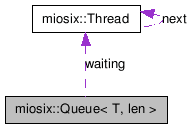# miosix::Queue< T, len > Class Template Reference [Syncronization]

`#include <sync.h>`

Collaboration diagram for miosix::Queue< T, len >:[legend]

List of all members.

## Public Member Functions

Queue ()
bool is_empty () const
bool is_full () const
void wait_until_not_empty ()
void wait_until_not_full ()
void get (T &elem)
void put (T elem)
bool IRQget (T &elem)
bool IRQget (T &elem, bool &hppw)
bool IRQput (T elem)
bool IRQput (T elem, bool &hppw)
void reset ()
void IRQreset ()

## Detailed Description

### template<typename T, unsigned int len> class miosix::Queue< T, len >

A queue, used to transfer data between TWO threads, or between ONE thread and an IRQ.
If you need to tranfer data between more than two threads, you need to use mutexes to ensure that only one thread at a time calls get, and only one thread at a time calls put.
This queue is meant to be a static or global class. Dynamically creating a queue with new or on the stack must be done with care, to avoid deleting a queue with a waiting thread, and to avoid situations where a thread tries to access a deleted queue.
Template Parameters:
 T the type of elements in the queue len the length of the Queue

## Constructor & Destructor Documentation

template<typename T, unsigned int len>
 miosix::Queue< T, len >::Queue ( ) ` [inline]`

Constructor, create a new empty queue.

## Member Function Documentation

template<typename T, unsigned int len>
 bool miosix::Queue< T, len >::is_empty ( ) const` [inline]`

Returns:
true if the queue is empty

template<typename T, unsigned int len>
 bool miosix::Queue< T, len >::is_full ( ) const` [inline]`

Returns:
true if the queue is full

template<typename T, unsigned int len>
 void miosix::Queue< T, len >::wait_until_not_empty ( ) ` [inline]`

If a queue is empty, waits until the queue is not empty.

template<typename T, unsigned int len>
 void miosix::Queue< T, len >::wait_until_not_full ( ) ` [inline]`

If a queue is full, waits until the queue is not full

template<typename T, unsigned int len>
 void miosix::Queue< T, len >::get ( T & elem ) ` [inline]`

Get an element from the queue. If the queue is empty, then sleep until an element becomes available.

Parameters:
 elem an element from the queue

template<typename T, unsigned int len>
 void miosix::Queue< T, len >::put ( T elem ) ` [inline]`

Put an element to the queue. If the queue is full, then sleep until a place becomes available.

Parameters:
 elem element to add to the queue

template<typename T, unsigned int len>
 bool miosix::Queue< T, len >::IRQget ( T & elem ) ` [inline]`

Get an element from the queue, only if the queue is not empty.
Can ONLY be used inside an IRQ, or when interrupts are disabled.

Parameters:
 elem an element from the queue. The element is valid only if the return value is true
Returns:
true if the queue was not empty

template<typename T, unsigned int len>
 bool miosix::Queue< T, len >::IRQget ( T & elem, bool & hppw ) ` [inline]`

Get an element from the queue, only if the queue is not empty.
Can ONLY be used inside an IRQ, or when interrupts are disabled.

Parameters:
 elem an element from the queue. The element is valid only if the return value is true hppw is not modified if no thread is woken or if the woken thread has a lower or equal priority than the currently running thread, else is set to true
Returns:
true if the queue was not empty

template<typename T, unsigned int len>
 bool miosix::Queue< T, len >::IRQput ( T elem ) ` [inline]`

Put an element to the queue, only if th queue is not full.
Can ONLY be used inside an IRQ, or when interrupts are disabled.

Parameters:
 elem element to add. The element has been added only if the return value is true
Returns:
true if the queue was not full.

template<typename T, unsigned int len>
 bool miosix::Queue< T, len >::IRQput ( T elem, bool & hppw ) ` [inline]`

Put an element to the queue, only if th queue is not full.
Can ONLY be used inside an IRQ, or when interrupts are disabled.

Parameters:
 elem element to add. The element has been added only if the return value is true hppw is not modified if no thread is woken or if the woken thread has a lower or equal priority than the currently running thread, else is set to true
Returns:
true if the queue was not full.

template<typename T, unsigned int len>
 void miosix::Queue< T, len >::reset ( ) ` [inline]`

Clear all items in the queue.
Cannot be used inside an IRQ

template<typename T, unsigned int len>
 void miosix::Queue< T, len >::IRQreset ( ) ` [inline]`

Same as reset(), but to be used only inside IRQs or when interrupts are disabled.

The documentation for this class was generated from the following file:
• /home/fede/Documents/Projects/ARM/miosix/miosix_np/miosix/kernel/sync.h

Generated on Fri Jun 19 15:19:04 2009 for Miosix by1.5.6